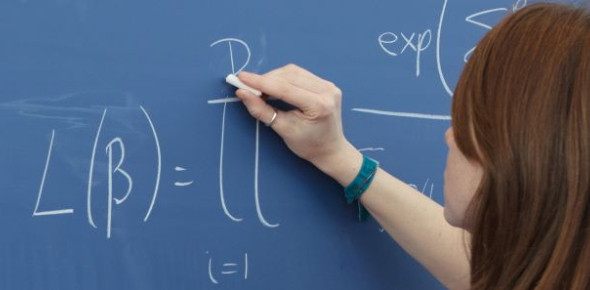# Principles Of Mathematics MCQ Quiz

9 Questions | Total Attempts: 11188SettingsMost people have a negative attitude when it comes to math, mostly because they do not allow themselves to give it a chance. Have you passed ninth grade and think you are good at math? If so, then the Principles of Mathematics MCQ Quiz is perfect to evaluate how much of it you understand; it won’t be so hard for you.

• 1.
What is the formula to determine the interior angles of a polygon?
• A.

N(-2-180)

• B.

-2(n+180)

• C.

(n-2)180

• D.

(180-2)n

• E.

None of the above

• 2.
What is the formula to determine the slope?
• A.

X=run/rise

• B.

M=rise/run

• C.

Run=m/rise

• D.

Rise+run=m

• E.

None of the above

• 3.
What is the total interior angle sum of a polygon?
• A.

180 degrees

• B.

90 degrees

• C.

360 degrees

• D.

270 degrees

• E.

Undefined

• 4.
What is the slope of this line, 6/0?
• A.

6

• B.

0

• C.

Undefined

• D.

1

• E.

None of the above

• 5.
6x-6x+4c+12-4c+12
• A.

12

• B.

24

• C.

0

• D.

6

• E.

1

• 6.
What is the formula for pythagoreon theorom?
• A.

2c=a+c

• B.

C=a+b

• C.

C=a-b

• D.

C=a*b

• E.

A=c/b

• 7.
How many quadrants are there in a Catesian Plane?
• A.

0

• B.

1

• C.

2

• D.

3

• E.

4

• 8.
How many sides does a decagon have?
• A.

4

• B.

6

• C.

8

• D.

10

• E.

12

• 9.
Six times a number divide it by two equals three times a number...
• A.

6/2n=3n

• B.

6n/2=3n

• C.

3n/6n=2

• D.

2-3n=6n

• E.

NONE OF THE ABOVE

Related TopicsBack to top